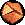# Stirling's Formula for N!

## Stirling's Formula

Remember N! = N (N-1) (N-2) ... 3 x 2 x 1? Stirling came up with a great formula:

N! ~ (2 Pi / (N+1))1/2E-(N+1) (N+1)(N+1)
(There is a more traditional, simpler formula, N! ~ (N/e)N (2 Pi N)1/2 which isn't quite as accurate; also, Bender and Orszag's formula extends the one I'm using.)

This formula is about 8% wrong for 1!, and 0.8% wrong for 10! - it gives better and better approximations for N! as N gets bigger and bigger. In fact, the fractional error becomes really close to 1/(12 N): if you multiply Stirling's formula by 1+1/(12 N) you get a formula which gets better and better even faster (with errors going as 1/N2). Bender and Orszag give an even better formula, involving z=N+1 as an expansion in bigger and bigger powers of z-1 = 1/z:

(z-1)! ~ (2 Pi / z)1/2E-z zz (1 +1/12 z-1 +1/288 z-2 -139/51840 z-3 -571/2488320 z-4 +163879/209018880 z-5 +5246819/75246796800 z-6 -534703531/902961561600 z-7 -4483131259/86684309913600 z-8 +...
What's the point? Well, it turns out that this fantastic formula does not converge. If you take 0!, the terms get smaller and smaller for a while, but the fifteenth term (according to Bender and Orszag) gets larger than 0.01, and the 35th term is bigger than 1010. Of course, the 35th term for 9! is 1010/1035 is really, really small - but the 175th term is bigger than one, and the 199th term is almost 1012.

This is typical of asymptotic series. An asymptotic series of length n approaches f(z) as z gets big, but for fixed z it can diverge as n gets larger and larger. This is still useful, but it isn't as stringent as the criteria for convergent series, which have to approach for each z as n gets large (as described, for example, in Matthews and Walker).

You might have noticed that we slipped something in back there. What is 0! (zero factorial), anyway? Well, you know that N! = N * (N-1)!, so (N-1)! = N! / N, and so 0! = 1. (Try it: 3! = 3 x 2 x 1 = 6; 2! = 2 = 3!/3, 1! = 1 = 2!/2, so 0! = 1!/1 = 1). Taking this further, we find out that (-1)! = 1/0 = infinity, (-2)! = (-1)! / -1 = -infinity, (-3)! = (-2)!/(-2) = infinity/2 = infinity ...

Thus N! is infinity whenever N is a negative integer. The fact that Stirling's formula for (z-1)! has zero radius of convergence as a series in 1/z is due to these infinities. A non-zero radius of convergence in 1/z would have to include some negative values near zero - hence some large negative integers. Since this factorial function (z-1)! (also known as the Gamma function) has infinities at all the negative integers, it can't be described by a convergent series there. It can't converge for 10! because it mustn't converge for (-12)!Statistical Mechanics: Entropy, Order Parameters, and Complexity, now available at Oxford University Press (USA, Europe).